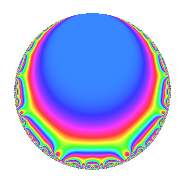Properties

 Label 4029.2.a.cLevel 4029 Weight 2 Character orbit 4029.a Self dual Yes Analytic conductor 32.172 Analytic rank 1 Dimension 2 CM No Inner twists 1

Related objects

Newspace parameters

 Level: $$N$$ = $$4029 = 3 \cdot 17 \cdot 79$$ Weight: $$k$$ = $$2$$ Character orbit: $$[\chi]$$ = 4029.a (trivial)

Newform invariants

 Self dual: Yes Analytic conductor: $$32.1717269744$$ Analytic rank: $$1$$ Dimension: $$2$$ Coefficient field: $$\Q(\sqrt{2})$$ Coefficient ring: $$\Z[a_1, a_2]$$ Coefficient ring index: $$1$$ Fricke sign: $$1$$ Sato-Tate group: $\mathrm{SU}(2)$

$q$-expansion

Coefficients of the $$q$$-expansion are expressed in terms of $$\beta = \sqrt{2}$$. We also show the integral $$q$$-expansion of the trace form.

 $$f(q)$$ $$=$$ $$q$$ $$+ \beta q^{2}$$ $$+ q^{3}$$ $$+ ( -1 + \beta ) q^{5}$$ $$+ \beta q^{6}$$ $$+ q^{7}$$ $$-2 \beta q^{8}$$ $$+ q^{9}$$ $$+O(q^{10})$$ $$q$$ $$+ \beta q^{2}$$ $$+ q^{3}$$ $$+ ( -1 + \beta ) q^{5}$$ $$+ \beta q^{6}$$ $$+ q^{7}$$ $$-2 \beta q^{8}$$ $$+ q^{9}$$ $$+ ( 2 - \beta ) q^{10}$$ $$-4 \beta q^{11}$$ $$+ \beta q^{14}$$ $$+ ( -1 + \beta ) q^{15}$$ $$-4 q^{16}$$ $$+ q^{17}$$ $$+ \beta q^{18}$$ $$+ ( -5 + 2 \beta ) q^{19}$$ $$+ q^{21}$$ $$-8 q^{22}$$ $$+ ( -3 + 3 \beta ) q^{23}$$ $$-2 \beta q^{24}$$ $$+ ( -2 - 2 \beta ) q^{25}$$ $$+ q^{27}$$ $$+ ( 2 + 2 \beta ) q^{29}$$ $$+ ( 2 - \beta ) q^{30}$$ $$+ ( 4 - 4 \beta ) q^{31}$$ $$-4 \beta q^{33}$$ $$+ \beta q^{34}$$ $$+ ( -1 + \beta ) q^{35}$$ $$+ ( -5 + 4 \beta ) q^{37}$$ $$+ ( 4 - 5 \beta ) q^{38}$$ $$+ ( -4 + 2 \beta ) q^{40}$$ $$+ ( 2 + 4 \beta ) q^{41}$$ $$+ \beta q^{42}$$ $$+ ( 2 - 6 \beta ) q^{43}$$ $$+ ( -1 + \beta ) q^{45}$$ $$+ ( 6 - 3 \beta ) q^{46}$$ $$+ ( -3 - 5 \beta ) q^{47}$$ $$-4 q^{48}$$ $$-6 q^{49}$$ $$+ ( -4 - 2 \beta ) q^{50}$$ $$+ q^{51}$$ $$+ ( -11 - \beta ) q^{53}$$ $$+ \beta q^{54}$$ $$+ ( -8 + 4 \beta ) q^{55}$$ $$-2 \beta q^{56}$$ $$+ ( -5 + 2 \beta ) q^{57}$$ $$+ ( 4 + 2 \beta ) q^{58}$$ $$+ ( -5 + \beta ) q^{59}$$ $$+ ( 5 - 4 \beta ) q^{61}$$ $$+ ( -8 + 4 \beta ) q^{62}$$ $$+ q^{63}$$ $$+ 8 q^{64}$$ $$-8 q^{66}$$ $$-10 q^{67}$$ $$+ ( -3 + 3 \beta ) q^{69}$$ $$+ ( 2 - \beta ) q^{70}$$ $$+ ( -6 - 5 \beta ) q^{71}$$ $$-2 \beta q^{72}$$ $$+ ( 2 - 9 \beta ) q^{73}$$ $$+ ( 8 - 5 \beta ) q^{74}$$ $$+ ( -2 - 2 \beta ) q^{75}$$ $$-4 \beta q^{77}$$ $$- q^{79}$$ $$+ ( 4 - 4 \beta ) q^{80}$$ $$+ q^{81}$$ $$+ ( 8 + 2 \beta ) q^{82}$$ $$+ ( 4 + \beta ) q^{83}$$ $$+ ( -1 + \beta ) q^{85}$$ $$+ ( -12 + 2 \beta ) q^{86}$$ $$+ ( 2 + 2 \beta ) q^{87}$$ $$+ 16 q^{88}$$ $$+ ( -12 + 4 \beta ) q^{89}$$ $$+ ( 2 - \beta ) q^{90}$$ $$+ ( 4 - 4 \beta ) q^{93}$$ $$+ ( -10 - 3 \beta ) q^{94}$$ $$+ ( 9 - 7 \beta ) q^{95}$$ $$+ ( 16 + \beta ) q^{97}$$ $$-6 \beta q^{98}$$ $$-4 \beta q^{99}$$ $$+O(q^{100})$$ $$\operatorname{Tr}(f)(q)$$ $$=$$ $$2q$$ $$\mathstrut +\mathstrut 2q^{3}$$ $$\mathstrut -\mathstrut 2q^{5}$$ $$\mathstrut +\mathstrut 2q^{7}$$ $$\mathstrut +\mathstrut 2q^{9}$$ $$\mathstrut +\mathstrut O(q^{10})$$ $$2q$$ $$\mathstrut +\mathstrut 2q^{3}$$ $$\mathstrut -\mathstrut 2q^{5}$$ $$\mathstrut +\mathstrut 2q^{7}$$ $$\mathstrut +\mathstrut 2q^{9}$$ $$\mathstrut +\mathstrut 4q^{10}$$ $$\mathstrut -\mathstrut 2q^{15}$$ $$\mathstrut -\mathstrut 8q^{16}$$ $$\mathstrut +\mathstrut 2q^{17}$$ $$\mathstrut -\mathstrut 10q^{19}$$ $$\mathstrut +\mathstrut 2q^{21}$$ $$\mathstrut -\mathstrut 16q^{22}$$ $$\mathstrut -\mathstrut 6q^{23}$$ $$\mathstrut -\mathstrut 4q^{25}$$ $$\mathstrut +\mathstrut 2q^{27}$$ $$\mathstrut +\mathstrut 4q^{29}$$ $$\mathstrut +\mathstrut 4q^{30}$$ $$\mathstrut +\mathstrut 8q^{31}$$ $$\mathstrut -\mathstrut 2q^{35}$$ $$\mathstrut -\mathstrut 10q^{37}$$ $$\mathstrut +\mathstrut 8q^{38}$$ $$\mathstrut -\mathstrut 8q^{40}$$ $$\mathstrut +\mathstrut 4q^{41}$$ $$\mathstrut +\mathstrut 4q^{43}$$ $$\mathstrut -\mathstrut 2q^{45}$$ $$\mathstrut +\mathstrut 12q^{46}$$ $$\mathstrut -\mathstrut 6q^{47}$$ $$\mathstrut -\mathstrut 8q^{48}$$ $$\mathstrut -\mathstrut 12q^{49}$$ $$\mathstrut -\mathstrut 8q^{50}$$ $$\mathstrut +\mathstrut 2q^{51}$$ $$\mathstrut -\mathstrut 22q^{53}$$ $$\mathstrut -\mathstrut 16q^{55}$$ $$\mathstrut -\mathstrut 10q^{57}$$ $$\mathstrut +\mathstrut 8q^{58}$$ $$\mathstrut -\mathstrut 10q^{59}$$ $$\mathstrut +\mathstrut 10q^{61}$$ $$\mathstrut -\mathstrut 16q^{62}$$ $$\mathstrut +\mathstrut 2q^{63}$$ $$\mathstrut +\mathstrut 16q^{64}$$ $$\mathstrut -\mathstrut 16q^{66}$$ $$\mathstrut -\mathstrut 20q^{67}$$ $$\mathstrut -\mathstrut 6q^{69}$$ $$\mathstrut +\mathstrut 4q^{70}$$ $$\mathstrut -\mathstrut 12q^{71}$$ $$\mathstrut +\mathstrut 4q^{73}$$ $$\mathstrut +\mathstrut 16q^{74}$$ $$\mathstrut -\mathstrut 4q^{75}$$ $$\mathstrut -\mathstrut 2q^{79}$$ $$\mathstrut +\mathstrut 8q^{80}$$ $$\mathstrut +\mathstrut 2q^{81}$$ $$\mathstrut +\mathstrut 16q^{82}$$ $$\mathstrut +\mathstrut 8q^{83}$$ $$\mathstrut -\mathstrut 2q^{85}$$ $$\mathstrut -\mathstrut 24q^{86}$$ $$\mathstrut +\mathstrut 4q^{87}$$ $$\mathstrut +\mathstrut 32q^{88}$$ $$\mathstrut -\mathstrut 24q^{89}$$ $$\mathstrut +\mathstrut 4q^{90}$$ $$\mathstrut +\mathstrut 8q^{93}$$ $$\mathstrut -\mathstrut 20q^{94}$$ $$\mathstrut +\mathstrut 18q^{95}$$ $$\mathstrut +\mathstrut 32q^{97}$$ $$\mathstrut +\mathstrut O(q^{100})$$

Embeddings

For each embedding $$\iota_m$$ of the coefficient field, the values $$\iota_m(a_n)$$ are shown below.

For more information on an embedded modular form you can click on its label.

Label $$\iota_m(\nu)$$ $$a_{2}$$ $$a_{3}$$ $$a_{4}$$ $$a_{5}$$ $$a_{6}$$ $$a_{7}$$ $$a_{8}$$ $$a_{9}$$ $$a_{10}$$
1.1
 −1.41421 1.41421
−1.41421 1.00000 0 −2.41421 −1.41421 1.00000 2.82843 1.00000 3.41421
1.2 1.41421 1.00000 0 0.414214 1.41421 1.00000 −2.82843 1.00000 0.585786
 $$n$$: e.g. 2-40 or 990-1000 Significant digits: Format: Complex embeddings Normalized embeddings Satake parameters Satake angles

Inner twists

This newform does not admit any (nontrivial) inner twists.

Atkin-Lehner signs

$$p$$ Sign
$$3$$ $$-1$$
$$17$$ $$-1$$
$$79$$ $$1$$

Hecke kernels

This newform can be constructed as the intersection of the kernels of the following linear operators acting on $$S_{2}^{\mathrm{new}}(\Gamma_0(4029))$$:

 $$T_{2}^{2}$$ $$\mathstrut -\mathstrut 2$$ $$T_{5}^{2}$$ $$\mathstrut +\mathstrut 2 T_{5}$$ $$\mathstrut -\mathstrut 1$$